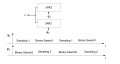# why timing diagram of combined SAR ADC is more efficient

#### yef smith

Joined Aug 2, 2020
291
Hello,In the timing diagram bellow we have one SAR sampling whi the other is proccesing.
If we have a stream of samples Wich is twicee as fast then the rate of each SAR.
given data stream of N+1,N,N-1,N-2
at first we give "N+1" to SAR1 and its proccesing it.
What happens of the second SAR,when do we give it the "N" sample?
As i see it we should give "N+1" to SAR1 and "N" to SAR2 simultaniosly.
but in the timing diagram bellow its not like that.
Why?
Thanks.#### Papabravo

Joined Feb 24, 2006
16,830
You can't give the samples simultaneously because they are not available simultaneously. They occur in a defined time sequence.

#### yef smith

Joined Aug 2, 2020
291
Hello Papabravo,What is the rate of convertion if we cant put the clocks exactly oposite to each other?
Thanks.

#### bogosort

Joined Sep 24, 2011
678
What is the rate of convertion if we cant put the clocks exactly oposite to each other?
Ask yourself this: How many clock steps does each ADC require to produce an output code for the corresponding value of Vin?

#### yef smith

Joined Aug 2, 2020
291
for a single its tsample+N_bit*switch.
So what happens if the clocks of the SAR's are not opposite to each other?
wy we cant double the rate of sampling proccessing.
Thanks.

Ask yourself this: How many clock steps does each ADC require to produce an output code for the corresponding value of Vin?

#### Papabravo

Joined Feb 24, 2006
16,830

•yef smith

#### bogosort

Joined Sep 24, 2011
678
for a single its tsample+N_bit*switch.
No idea what you mean by this. Try to stick to the shared terminology in the image you provided.

Consider each ADC separately. For the top ADC, on the first clock step the ADC samples the voltage at Vin; on the second clock step, it performs a binary search to produce an output code. If we assume that both the sample and hold stage and the binary search stage each take one clock step, how many clock steps does it take to produce a sample value from a voltage? The answer is two clock steps. Do you see that?

The bottom ADC requires the same number of clock steps as the top ADC. Do you see that?

Suppose the clock produces two clock steps in one second. How many samples will the top ADC produce in one second? Well, it takes two clock steps to produce a sample, and there are two clock steps per second, so the top ADC will produce one sample per second.

What about the bottom ADC? On its own, it will also produce one sample per second (which makes sense, as it's the same as the top ADC).

Now, let's connect the two ADCs to the same input, as in the image you posted. At time t = 0 seconds, the top ADC is in the sample and hold stage, which means that's it connected to the input and reading what will become sample N. At time t = 0, the bottom ADC is in the binary search stage, which means that's it not connected to the input; instead, it's in the middle of producing an output code for what will become sample N + 1.

At time t = 0.5, the top ADC is disconnected from the input and doing a binary search for sample N. At time t = 0.5, the bottom ADC has finished with sample N + 1 and is now reading the input for sample N - 1.

At time t = 1, the top ADC has finished with sample N and is now reading the input for sample N - 2. At time t = 1, the bottom ADC is disconnected from the input and is binary searching for the code for sample N - 1.

In one second, the system produced two samples: N + 1 (by the bottom ADC) and N (by the top ADC). In the next second, the system will produce two more samples: N - 1 (by the bottom ADC) and N - 2 (top ADC), and so on.

Since we originally determined that a single ADC produces one sample per second, we see that two ADCs with opposite timing doubles the rate.

So what happens if the clocks of the SAR's are not opposite to each other?
wy we cant double the rate of sampling proccessing.
Hopefully you can now work out why the opposite timing is required. As a test for yourself, can you describe what would happen to the sequence of samples if both ADCs used the same clock timing?

#### RPLaJeunesse

Joined Jul 29, 2018
183
Your diagram implies that each SAR has one clock. Not the case. There is a sample clock, and there is a conversion clock. Yes, the conversion clock may be internal, and it may thus be free-running. But it is the sample clock, which occurs once per sample, that initiates the the conversion process. As long as the two identical SARs have their sample clocks exactly 180 degrees out of phase the desired interleaved sampling will occur. Needless to say the sample clocks need to be slow enough that all the needed conversion clock cycles occur in each sample interval.